Spitzer Documentation & Tools
IRS Instrument Handbook

### 5.1.14         SLOPE_FINDER

The SLOPE_FINDER module converts a 3-dimensional data cube (ramp signal vs. time) to a 2-dimensional image containing slope-estimated signal intensities (electron/sec). The estimation of ramp slopes consists of the following algorithm. Note that all pixels are fit independently.

First, problematic samples are identified. These samples are excluded from the ramp fitting. In the IRS pipelines the first sample of every 8- or 16-sample ramp is excluded. Other excluded samples are: pixels masked in the pmask (the entire ramp is deemed unusable and the output slope is NaN); samples having the following dmask bits: radhits (bit # 9), as well as all post-radhit samples in the same pixel; saturated samples, (bit # 3); samples with “data missing” (bit # 14); and samples that were uncorrectable for non-linearity (bit # 12 or 13).

After discarding problematic samples, arrays of the remaining samples are constructed: pi and ni are the sample value and plane number for the ith sample in these arrays, where i = 1...N and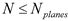. (Nplanes is the original number of samples or planes in the DCE ramps, and N is the number of useful or non-problematic samples).

If N < 2, the output slope is NaN. Else, the slopes between consecutive useful samples and their average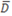are computed: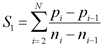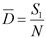Equation 5.12

The standard deviation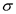of this average slope is obtained from: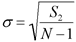Equation 5.13

Individual slopes (pi-pi-1)/(ni-ni-1) are compared with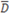, and if the worst one differs from it by more than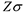, it is rejected. The parameter Z, also referred to as “Sigma Rejection Factor,” has a value of 2.0. The slope averaging procedure, namely the computation of S1, S2,, and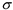, may be repeated, this time excluding rejected slopes, and yielding in turn additional rejected slopes. However, the procedure is done only once. That is, the parameter “Maximum # Rejection Iterations,” which is the number of times the slope averaging and rejection procedure is executed, has a default value of one.

After the above sequence, the ramp is broken into segments, each consisting of two or more consecutive non-problematic samples. Segment breaks are defined by rejected slopes in the slope-averaging sequence. The slope of each segment and its uncertainty is computed by a linear fit, using the procedure described in Appendix A of Gordon et al. 2005, P.A.S.P., 117, 503–525, “Reduction Algorithms for the Multi-band Imaging Photometer for Spitzer.” The algorithm takes into account the correlation of uncertainties of the input samples, and it propagates them throughout.

The weighted mean of the various segment slopes (weighted by the inverse variance) is the final slope of the ramp. This slope and its uncertainty are multiplied by Nplanes to obtain the “top-plane” signal and its, and divided by the integration time of the DCE. The resulting outputs are slopes in units of electron/sec.Figure 5.7  Slope in the presence of a radhit: the radhit shown occurs in sample # 8 of the ramp. SLOPE_FINDER excludes it and subsequent (top) samples, because the slope after a radhit is shallower than the slope extrapolated (dashed line) from the bottom of the ramp. The output slope (solid line) is computed from the bottom samples preceding the radhit. Sample # 1 is always excluded, and the slope between samples # 4 and 5 in this ramp is excluded as an outlier, during the outlier rejection iteration. Shown also are slopes ±1from the mean slope, whereis the output uncertainty for this ramp.